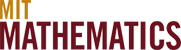# 18.2x and 18.3x

## Applied Mathematics

This heading includes discrete applied mathematics (18.2x), including Combinatorics, as well as continuous applied mathematics (18.3x). The gateway subjects to these two areas are:

• 18.200 (Principles of Discrete Applied Mathematics) develops the principles of discrete applied mathematics. It carries CI-M credit. Prerequisites: 18.02. The variant, 18.200A, covers the same material as 18.200, except for the communication-intensive component and does not give CI-M credit.

• 18.300 (Principles of Continuum Applied Mathematics) develops the principles of continuous applied mathematics. Prerequisites: 18.02 and 18.03.

Other basic subjects include:

• 18.211 (Combinatorial Analysis) is a foundational subject in combinatorics. Prerequisites: 18.02 and Linear Algebra.

• 18.303 (Linear Partial Differential Equations) develops the classical partial differential equations of applied mathematics. Prerequisite: Linear Algebra.

• 18.330 (Introduction to Numerical Analysis) develops the foundations of numerical analysis. Prerequisites: 18.02 and 18.03.

Further applied mathematics subjects, requiring somewhat more background, include:

• 18.212 (Algebraic Combinatorics) a more algebraically demanding approach to combinatorics than 18.211. Prerequisites: 18.701 or 18.703.

• 18.353J (Nonlinear Dynamics: Chaos) coincides with 2.050J and with 12.006J. Prerequisites: 18.03 and 8.02.

• 18.354J (Nonlinear Dynamics: Continuum Systems) coincides with 1.062J and 12.207J, and serves as a partner to 18.353. Prerequisites: 18.03 and 8.02.

There are two Undergraduate Seminars in applied mathematics:

• 18.204 (Undergraduate Seminar in Discrete Mathematics) Prerequisites: 18.200 or 18.062; and Linear Algebra.

• 18.384 (Undergraduate Seminar in Physical Mathematics) focusing on continuous applied mathematics. Prerequisites: 18.300 or 18.353 or 18.354.